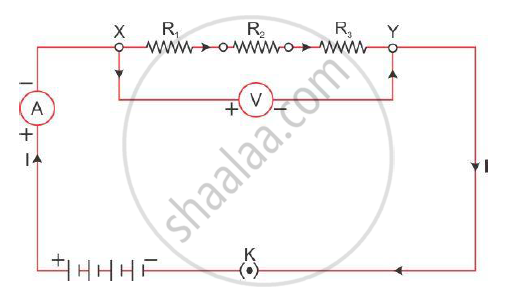SSC (Marathi Semi-English) 10thMaharashtra State Board
Share

# Find the Expression for Resistors Connected in Series. - SSC (Marathi Semi-English) 10th - Science and Technology - 1

ConceptResistance of a System of Resistors - Resistors in Series

#### Question

Find the expression for resistors connected in series.

#### Solution

When two or more resistors are joined from end to end, the resistances are connected in series.The current in series remains the same across all the resistors.

The potential difference is the sum of potential differences across all the individual resistors.

V = V1 + V2 + V3                                                                                       … (1)

Let I be the current in the circuit.

On applying Ohm’s law to the entire circuit, we get

V = IRs … (2)

Here, Rs is the combined resistance of the circuit.

Now, applying Ohm’s law to individual resistances, we get

V1 = IR1

V2 = IR2

V3 = IR3                                                                                       ......(3)

From equations (1), (2) and (3), we get

IRs = IR1 + IR2 + IR3

∴ Rs = R1 + R2 + R3

Here, Rs is the resultant resistance. Thus, the resultant resistance of a series combination of resistors is the sum of individual resistances.

The resultant resistance is greater than all the resistances.

Is there an error in this question or solution?

#### APPEARS IN

2012-2013 (March) (with solutions)
Question 4.2 | 5.00 marks
Solution Find the Expression for Resistors Connected in Series. Concept: Resistance of a System of Resistors - Resistors in Series.
S